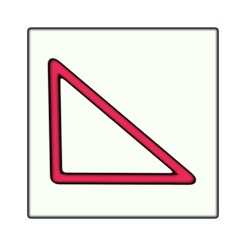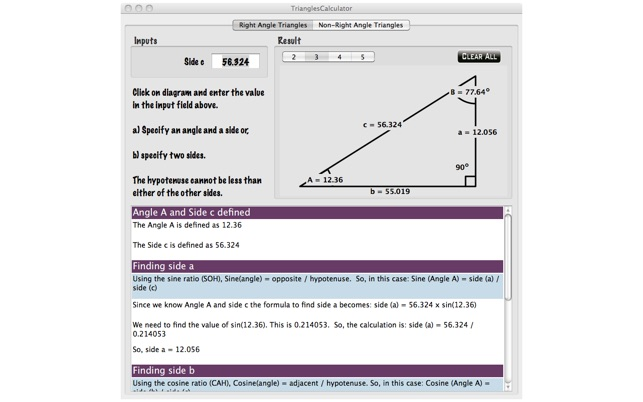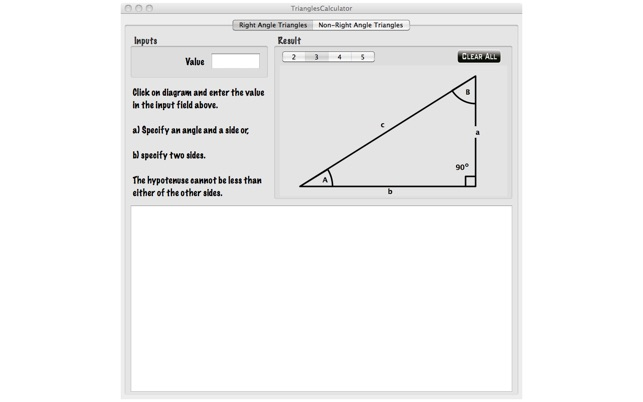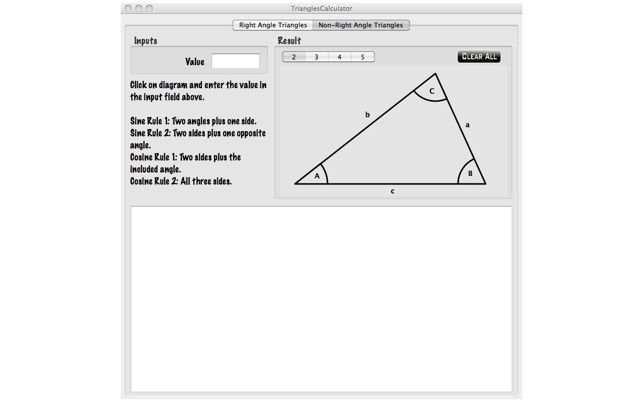## Screenshots

••••## Description

Do you need to learn how to determine the angles and lengths of a Right Angle Triangle? Need to clearly understand which of the sine, cosine and tangent ratios to use? Need to know how to determine the angle when you know two of the sides. If the answer is Yes to any of these questions then this App is for you. The Angles Calculator not only calculates the answers for you but it also tells you how it did it so you can understand how and when to apply the rules for right angle triangles.

Now that you know right angled triangles what about non-right-angled triangles? It all the same problems but with different formula to apply to calculate the unknown sides and angles.

The App is made up of two parts each with a diagram - one part for right angled triangles and one for non-right angled triangles. The Tab at the top allows you to select which one you want to do.

To specify known parts of the triangle click on the side or angle on the diagram that you know. The label at the top left will change to specify that side or angle. You can now use the keyboard to enter the value. Press enter and the value will be used to update the diagram. You now click on the diagram to select the next value. The label to the left changes to show that it is this value to be specified, and so on.

For both right angled triangles or non-right angled triangles once you have specified enough information for the calculations to be done then the answers appear on the diagram and a full explanation is given below to explain how the answers were arrived at.

If you have specified invalid information then the diagram will update to show this information but none of the unknown sides or angles will be calculated. The explanation text will explain in a red row what was wrong. You can then correct the problem and the answers should then be shown.

To start over just select 'Clear All'. All values are reset ready for you to enter new values.

## What’s New

Version 1.1.1

Bug fix in the Angles Calculator. Rounding errors.

## Information

Seller
Jim Chapple
Size
436.8 KB
Category
Education
Compatibility

OS X 10.6 or later, 64-bit processor

Languages

English

Age Rating
Rated 4+
•